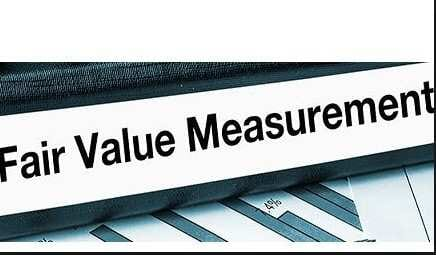# Cash-flow-based measurement techniques

Fair value is many times determined indirectly using measurement techniques, forexample, cash-flow-based measurement techniques, reflecting all the following factors:

1. estimates of future cash flows. Cash-flow-based measurement techniques
2. possible variations in the estimated amount or timing of future cash flows for the asset or liability being measured, caused by the uncertainty inherent in the cash flows.
3. the time value of money. Cash-flow-based measurement techniques
4. the price for bearing the uncertainty inherent in the cash flows (a risk premium or risk discount). The price for bearing that uncertainty depends on the extent of that uncertainty. It also reflects the fact that investors would generally pay less for an asset (and generally require more for taking on a liability) that has uncertain cash flows than for an asset (or liability) whose cash flows are certain.
5. other factors, for example, liquidity, if market participants would take those factors into account in the circumstances.

Cash-flow-based measurement techniques are not measurement bases. They are techniques used in applying a measurement basis. Hence, when using such a technique, it is necessary to identify which measurement basis is used and the extent to which the technique reflects the factors applicable to that measurement basis. For example, if the measurement basis is fair value, the applicable factors are those described above.

Cash-flow-based measurement techniques can be used in applying a modified measurement basis, for example, fulfilment value modified to exclude the effect ofthe possibility that the entity may fail to fulfill a liability (own credit risk). Modifying measurement bases may sometimes result in information that is more relevant to the users of financial statements or that may be less costly to produce or to understand. However, modified measurement bases may also be more difficult for users of financial statements to understand.

Outcome uncertainty arises from uncertainties about the amount or timing of future cash flows. Those uncertainties are important characteristics of assets and liabilities. When measuring an asset or liability by reference to estimates of uncertain future cash flows, one factor to consider is possible variations in the estimated amount or timing of those cash flows (see above, sub (b)). Those variations are considered in selecting a single amount from within the range of possible cash flows. The amount selected is itself sometimes the amount of a possible outcome, but this is not always the case. The amount that provides the most relevant information is usually one from within the central part of the range (a central estimate). Different central estimates provide different information. For example: Cash-flow-based measurement techniques

1. the expected value (the probability-weighted average, also known as the statistical mean) reflects the entire range of outcomes and gives more weight to the outcomes that are more likely. The expected value is not intended to predict the ultimate inflow or outflow of cash or other economic benefits arising from that asset or liability.
2. the maximum amount that is more likely than not to occur (similar to the statistical median) indicates that the probability of a subsequent loss is no more than 50% and that the probability of a subsequent gain is no more than 50%. Cash-flow-based measurement techniques
3. the most likely outcome (the statistical mode) is the single most likely ultimate inflow or outflow arising from an asset or liability.

A central estimate depends on estimates of future cash flows and possible variations in their amounts or timing. It does not capture the price for bearing the uncertainty that the ultimate outcome may differ from that central estimate (that is, the factor described in (see above, sub (d). Cash-flow-based measurement techniques

No central estimate gives complete information about the range of possible outcomes. Hence users may need information about the range of possible outcomes.